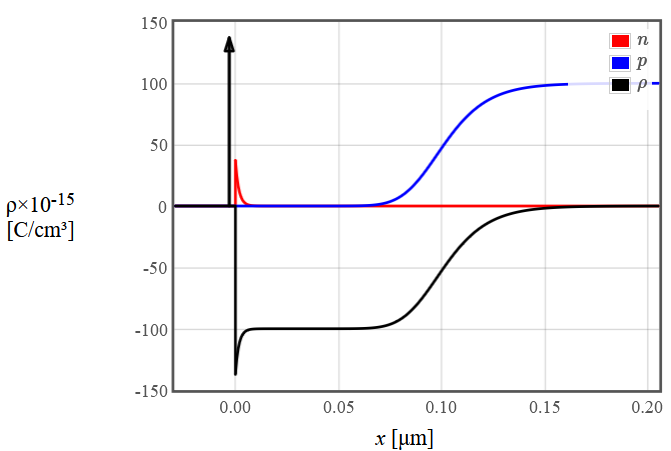PHT.301 Physics of Semiconductor Devices

## Subthreshold current

Consider a MOSFET biased in saturation so that the channel is pinched off near the drain. If the gate voltage is brought below the threshold voltage, the gradual channel approximation predicts that the drain current goes to zero but a small subthreshold current still flows in this regime. Just below the threshold voltage in an n-channel MOSFET, the electron concentration is highest next to the oxide near the source. This is the weak inversion regime and the electron density decays approximately exponentially as the distance increases from the oxide. This is illustrated in the charge density plot below.The electron density will be much lower near the drain and a diffusion current will flow as electrons diffuse from a high concentration near the source to a low concentration near the drain. An estimate for this diffusion current can be made using the depletion approximation. In this approximation, the subthreshold current is,

$$I_D \approx \frac{n_i^2\mu \epsilon_sk_B^2T^2W}{e^2N_A^2x_pL}\exp\left(\frac{e^2N_Ax_p^2}{2\epsilon_sk_BT}\right)$$

where $x_p$ is the depletion width,

$$x_p = -\frac{\epsilon_s}{\epsilon_{\text{ox}}}t_{\text{ox}} + \sqrt{\left( \frac{\epsilon_s}{\epsilon_{\text{ox}}}t_{\text{ox}} \right)^2 + \frac{2\epsilon_s}{eN_A}(V_g - V_{fb})}.$$

See the depletion approximation page for more details. The form below will calculate the subthreshold current for an n-channel MOSFET in the depletion approximation from weak inversion to strong inversion.

 $t_{ox}$ = nm $\epsilon_{ox}$ = $N_c(300)$ = 1/cm³ $T$ = K $E_g$ = eV $\epsilon_s$ = $N_v(300)$ = 1/cm³ $N_A$ = 1/cm³

$W$ =  m,  $L$ =  m,   $\mu$ =  cm²/Vs

 $V_{fb}$ =

$E_g=$  eV,  $n_i=$  1/cm³, $V_T=$  V

 $\log (I_D)$ A $V_g$ [V]

By operating a MOSFET in the subthreshold region a low power consumption can be realized. This is important for portable devices, IoT (Internet of Things) devices, wearable electronics, and battery-operated systems.